Function Repository Resource:

Create a beta distribution using the mean and a measure of its spread as parameters instead of using the conventional parameters

Contributed by: Seth J. Chandler
 ResourceFunction["MeanSpreadBetaDistribution"][mean,spread] creates a beta distribution that is centered at mean and with standard deviation of spread multiplied by the maximum possible standard deviation for mean.

## Details and Options

The mean and spread must both lie between 0 and 1.

## Examples

### Basic Examples (2)

Create a beta distribution whose mean is 2/3 and whose standard deviation is 1/2 of the maximum possible value given that mean:

 In:=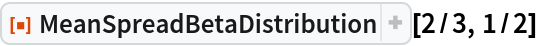Out=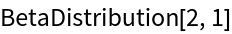Create a beta distribution whose mean is 1/4 and whose standard deviation is 9/10 of the maximum possible value given that mean:

 In:=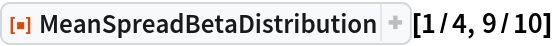Out=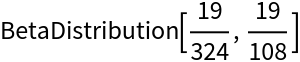### Scope (1)

The function handles symbolic parameters:

 In:=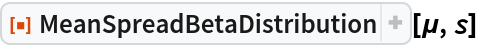Out=### Applications (1)

Show how decreasing the spread of a beta distribution affects the associated PDF :

 In:=Out=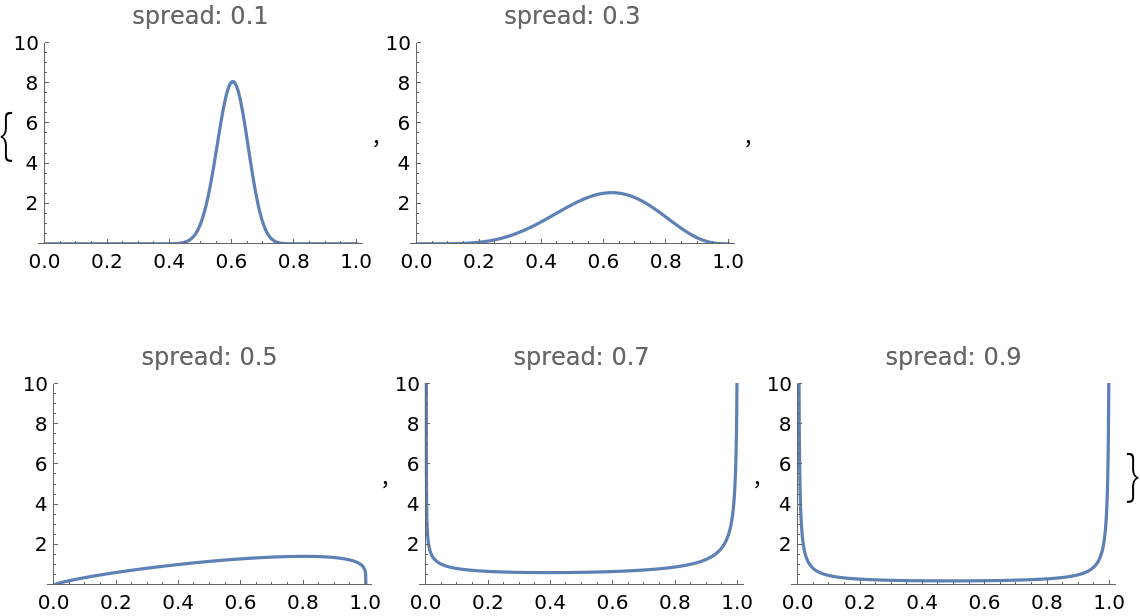### Properties and Relations (2)

One can take the parameters of a beta distribution and compute their mean and spread:

 In:=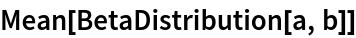Out=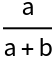In:=Out=Thus, for BetaDistribution[3,4] the mean is 3/7 and the spread is calculated below:

 In:=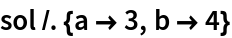Out=### Possible Issues (1)

If one uses a spread less than zero, the result is a meaningless beta distribution. With a value of greater than one, the result is a meaningless beta distribution with an illegal negative first parameter:

 In:=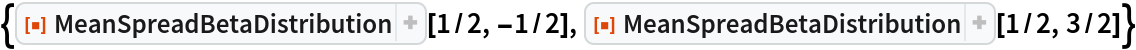Out=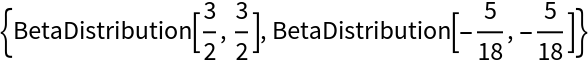Seth J. Chandler

## Version History

• 1.0.0 – 17 December 2019

## Author Notes

The reparameterization of the beta distribution is found by first computing a value σ[μ] that constitutes the maximum permissible standard deviation for a BetaDistribution with a given mean μ and then executing the following code, which solves for conventional parameters a and b: